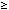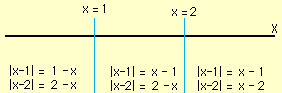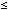Sender: Austin Cline

Solve for x: The absolute value of x-1 is less than or equal to the absolute value of x-2

Hi Austin,

To do this you need to think about the meaning of absolute value.

 |y| = y if y0 |y| = -y if y < 0

That is if y < 0 then change its sign by multiplying by -1.
Thus

 |x - 1| = x - 1 if x - 10 (that is x1) |x - 1| = -(x - 1) if x - 1 < 0 (that is x < 1)

and

 |x - 2| = x - 2 if x - 20 (that is x2) |x - 2| = -(x - 2) if x - 2 < 0 (that is x < 2)

Hence you need to divide the line into three pieces, by cutting it at x = 1 and x = 2, and solve the equation in each piece.• If x < 1 then |x - 1||x - 2| if and only if 1 - x2 - x, that is if and only if 12. But 1 is less than 2 so |x - 1||x - 2| is true for all x < 1.

• If 1x < 2 then |x - 1||x - 2| if and only if x - 12 - x, that is if and only if x3/2.

• If 2x then |x - 1||x - 2| if and only if x - 1x - 2, that is if and only if -1-2. Since -1 is not less than or equal to -2, |x - 1||x - 2| is false for all x satisfying 2x.

Thus |x - 1||x - 2| if and only if x3/2.

Cheers,
Harley

Go to Math Central